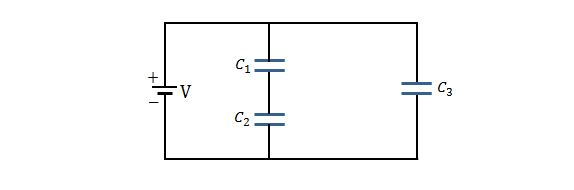Electricity and Magnetism

# Energy stored in a capacitor

Consider a parallel-plate capacitor with a plate area of $1.90\text{ cm}^2$ and the separation between two plates of $3.00\text{ mm}.$ It is charged fully by a $8.00\text{ V}$ battery and then disconnected from the battery. Then approximately how much work is required to pull apart the plates to a separation of $7.00\text{ mm}?$

The value of the permittivity constant is $\varepsilon_0=8.85 \times 10^{-12} \text{ C}^2\text{/N}\cdot\text{m}^2.$

If a parallel-plate capacitor with plate area $40\text{ cm}^2$ and plate spacing $1.2\text{ mm}$ is charged to a potential difference of $500\text{ V},$ how much energy is stored in the capacitor?

The value of the permittivity constant is $\varepsilon_0=8.85 \times 10^{-12} \text{ C}^2\text{/N}\cdot\text{m}^2.$

If two capacitors with respective capacitances $1.0\,\mu\text{F}$ and $4.0\,\mu\text{F}$ are connected in parallel across a $300\text{ V}$ potential difference, how much total energy is stored in the two capacitors?

What capacitance is required to store an energy of $8\text{ kW}\cdot\text{h}$ at a potential difference of $1000\text{ V}?$In the above circuit, the potential difference across the capacitor arrangement is $V=100\text{ V}$ and the capacitance are $C_1=10.00\,\mu\text{F}, C_2=5.00\,\mu\text{F}, C_3=2.00\,\mu\text{F}.$ Approximately how much energies are stored for the capacitors $1 (U_1$) and $2 (U_2$), respectively?

×